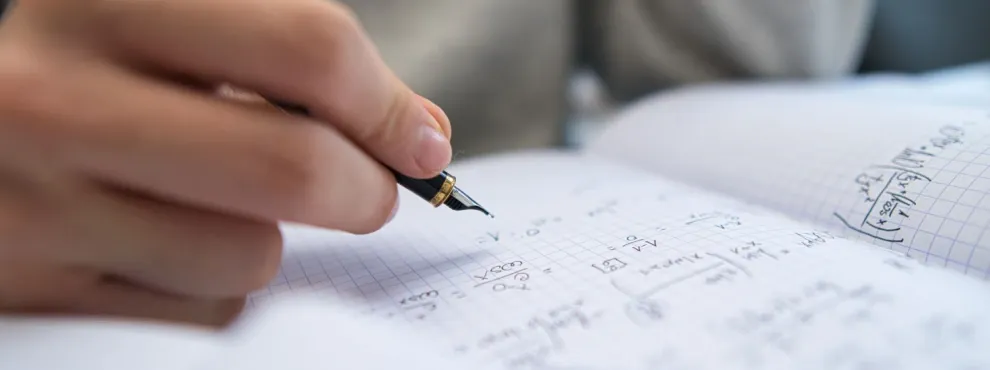Universities

# SRM University CGPA to Percentage Calculator

By Edvoy• Last updated: Oct 18, 2023Explore, filter, shortlist universities with ease and get free counselling

SRM University follows a CGPA (Cumulative Grade Point Average) system for evaluating the academic performance of its students. However, for various purposes, such as higher studies, scholarships, or job applications, students may need to convert their CGPA into a percentage. This article will discuss how to use the CGPA to percentage calculator, a handy tool for converting CGPA to percentage.

## SRM University CGPA to Percentage Conversion Formula

To convert SRM University CGPA to percentage using the formula of multiplying CGPA by 10, we need to use the following formula:

Percentage of Marks = CGPA x 10

This means that you need to multiply your CGPA by 10 to obtain the required percentage. For example, if your CGPA is 8.0, your percentage of marks would be 80% (8.0 x 10).

### SRM CGPA to Percentage Calculator

Explore, filter, shortlist universities with ease and get free counselling

## SRM University CGPA to Percentage Calculator in Engineering

Several online CGPA to percentage calculators can help you convert your CGPA into percentage quickly and accurately. Check out the calculator below to convert your CGPA into percentage quickly and efficiently.

It is essential to note that the CGPA to percentage calculator uses the formula of multiplying the CGPA by 10 to calculate the percentage of marks.

## SRM University CGPA to Percentage Conversion Certificate

Students may need to obtain a conversion certificate from SRM University to prove their academic performance in percentage terms. To get the conversion certificate, students need to follow the below-mentioned steps:

• Visit the official website of SRM University.
• Fill in the required details, such as name, roll number, department, and contact information.
• Attach a copy of your mark sheet or transcript.
• Submit the application form to the Controller of Examinations.
• Pay the prescribed fee, if any, to obtain the conversion certificate.
• It is essential to note that the conversion certificate is issued only to students who have completed their degree program successfully.

In conclusion, the SRM University CGPA to percentage calculator is a handy tool for converting CGPA to percentage. It uses the formula of multiplying CGPA by 10 to calculate the percentage of marks. Students can also obtain a conversion certificate from SRM University for various purposes. It is advisable to check the correct formula and procedures for CGPA to percentage conversion with the university.

Related pages,

## FAQ’s

### Q. What is CGPA?

1. CGPA stands for Cumulative Grade Point Average, which is an average of the grade points earned in all the courses or semesters of a program.

### Q. Why do we need to convert CGPA to percentage?

1. CGPA is a relative grading system that represents a student's academic performance on a scale of 10. However, some universities, colleges, or employers may require a percentage of marks for admission, scholarships, or job applications.

### Q. What is the formula for converting SRM University CGPA to percentage?

1. The formula for converting SRM University CGPA to percentage is CGPA x 10.

### Q. Can I use the same formula to convert CGPA to percentage for other universities?

1. The formula for converting CGPA to percentage may vary from university to university. Therefore, checking with the respective educational institution for the correct formula and procedures for CGPA to percentage conversion is advisable.
Explore, filter, shortlist universities with ease and get free counselling

### Q. Can I obtain a conversion certificate from SRM University for CGPA to percentage conversion?

1. Yes, students can obtain a conversion certificate from SRM University for various purposes. Students need to download the application form for conversion of CGPA to percentage, fill in the required details, attach a copy of their mark sheet or transcript, and submit the application form to the Controller of Examinations with the prescribed fee.

### Q. Is CGPA the same as percentage?

1. No, CGPA and percentage are not the same. CGPA is a relative grading system, while percentage is an absolute grading system. CGPA represents a student's academic performance on a scale of 10, while the percentage represents the marks obtained out of 100.

Start your journey and begin a conversation with a friendly Edvoy Counsellor and download the app today.

Related tags:
0 Likes